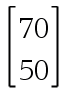6156 Predicting Transition Matrices

3 Questions | Total Attempts: 63SettingsIn this quiz, you are required to write down the responses to question where the answer is a matrix. For a matrix , write your answer 70, 50 (comma delimited, with a space). Round to the nearest whole number if required.

• 1.
For the transition matrix and the initial state matrix above, determine the value of .
• 2.
For the transition matrix and the initial state matrix above, determine the value of .
• 3.
For the transition matrix and the initial state matrix above, determine the value of .
• 4.
For the transition matrix and the initial state matrix above, determine the value of .
• 5.
For the transition matrix and the initial state matrix above, determine the value of .
• 6.
For the transition matrix and the initial state matrix above, determine the value of .
• 7.
For the transition matrix and the initial state matrix above, determine the value of .
• 8.
For the transition matrix and the initial state matrix above, determine the value of .
• 9.
For the transition matrix and the initial state matrix above, determine the value of .
• 10.
For the transition matrix and the initial state matrix above, determine the value of .
• 11.
For the transition matrix and the initial state matrix above, determine the value of .
• 12.
For the transition matrix and the initial state matrix above, determine the value of .
Related TopicsBack to top